## General Question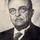# Trying to understand the magical number of e?

Asked by inunsure (423) November 7th, 2011

I’m getting a little confused with how this book explains e.

They say the derivative of 2^x when x=0 is 0.693, umm maybe I’m really dumb but when I try this with wolfram alpha I get 0

http://www.wolframalpha.com/input/?i=d%2Fdx+2%5E0+

Observing members: 0Composing members: 0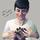The derivative of a^x (where a is a constant) is (a^x)ln(a). So for 2^x at x=0, your derivative is (2^0)ln(2) = .693.

Mariah (25863)“Great Answer” (4) Flag as…Thanks for the reply. I’m going to go back over what I read but that seems different that what I have just been doing with derivatives, could you try and give me a more intuitive sense of why you are timing it by the ln(x)

I’m going to see if I can understand as well

inunsure (423)“Great Answer” (0) Flag as…I dont have an intuitive sense of how to work out d/dx N^2, I think that’s what maybe holding me back.

inunsure (423)“Great Answer” (0) Flag as…Okay, here’s how I arrived at that derivative:

y = a^x
Take the natural log of both sides. Using the power rule, x goes out front.
ln(y) = x*ln(a)
Now use implicit differentiation (let me know if you want me to explain this step more thoroughly)
1/y dy = ln(a) dx
dy/dx = y*ln(a)
But we already established that y = a^x, so…
dy/dx = (a^x)ln(a)

This makes sense because for an exponential equation in which your base is e (a=e), the derivative is just e^x. You do it the same way and get (e^x)ln(e), but as ln(e) = 1, it goes down to just e^x.

Mariah (25863)“Great Answer” (4) Flag as…Do you want to see a proof of the derivative of n^2, too?

Mariah (25863)“Great Answer” (0) Flag as…No thats ok

inunsure (423)“Great Answer” (0) Flag as…I went through it with no problems I’ll just keep going over it

inunsure (423)“Great Answer” (0) Flag as…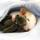You can also use the chain rule. For positive a, a^x is exp(x*ln(a)) by definition. (Writing exp() for e^ makes it easier to see whats going on):

(exp(x*ln(a)))’ = exp’(x*ln(a))*(x*ln(a))’.

Since exp is its own derivative and (x*ln(a))’ = ln(a),

(exp(x*ln(a)))’ = exp(x*ln(a))*ln(a) = ln(a)*a^x.

x^2, on the other hand, is x*x. By the product rule,

(x^2)’ = x*x’ + x’*x = x*1 + 1*x = 2x.

ratboy (15157)“Great Answer” (2) Flag as…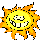Wow. This question is SO out of my field of expertise. I admire you who can think this way enormously!

Sunny2 (18810)“Great Answer” (0) Flag as…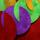I can’t help you, but maybe The Derivative Rag can. The “meat” of the video starts in at about 47 or really, the real meat starts at about 1 minute, 10 seconds. Anyway, it’s fun, if nothing else.

and since I’ve been on a Tom Lehrer kick lately, I will also post the Derivative Song. Don’t forget the “carefully.” :-)

lillycoyote (24835)“Great Answer” (1) Flag as…or